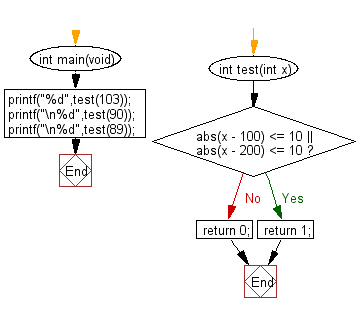﻿ C Program: Check an integer is within 10 of 100 or 200# C Exercises: Check a given integer and return true if it is within 10 of 100 or 200

## C-programming basic algorithm: Exercise-4 with Solution

Write a C program to check a given integer and return true if it is within 10 of 100 or 200.

C Code:

``````#include <stdio.h>
#include <stdlib.h>
int main(void){
printf("%d",test(103));
printf("\n%d",test(90));
printf("\n%d",test(89));
}
int test(int x)
{
if(abs(x - 100) <= 10 || abs(x - 200) <= 10)
return 1;
return 0;
}
``````

Sample Output:

```1
1
0
```

Flowchart:C Programming Code Editor:

What is the difficulty level of this exercise?

Test your Programming skills with w3resource's quiz.

﻿

## C Programming: Tips of the Day

How does array  = {0} set the entire array to 0?

The behavior of this code in C is described in section 6.7.8.21 of the C specification (online draft of C spec): for the elements that don't have a specified value, the compiler initializes pointers to NULL and arithmetic types to zero (and recursively applies this to aggregates).

The behavior of this code in C++ is described in section 8.5.1.7 of the C++ specification (online draft of C++ spec): the compiler aggregate-initializes the elements that don't have a specified value.

Also, note that in C++ (but not C), you can use an empty initializer list, causing the compiler to aggregate-initialize all of the elements of the array:

`char array = {};`

As for what sort of code the compiler might generate when you do this, take a look at this question: Strange assembly from array 0-initialization

Ref : https://bit.ly/3yxvK5c